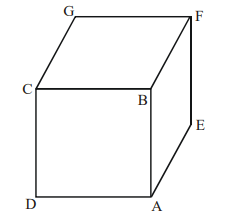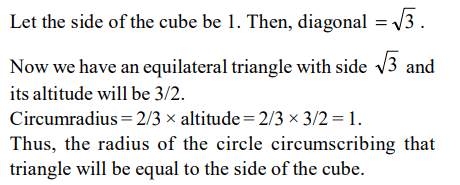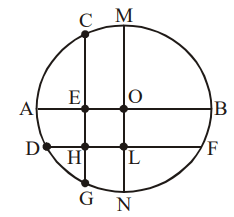## Geometry Questions and Answers Part-4

1. In the figure (not drawn to scale) given below, P is a point on AB such that AP : PB = 4 : 3. PQ is parallel to AC and QD is parallel to CP. In $\triangle ARC ,\angle ARC =90^{0}$    , and in $\triangle PQS, \angle PSQ =90^{0}$    . The length of QS is 6 cms. What is ratio AP : PD?a) 10 : 3
b) 2 : 1
c) 7 : 3
d) 8 : 3

Explanation:2. In the figure (not drawn to scale) given below, if AD = CD = BC, and $\angle BCE =96^{0}$    , how much is $\angle DBC$a) 32°
b) 84°
c) 64°
d) Cannot be determined.

Explanation:3.In the figure given below (not drawn to scale), A, B and C are three points on a circle with centre O. The chord BA is extended to a point T such that CT becomes a tangent to the circle at point C. If $\angle ATC =30^{0}$   and $\angle ACT =50^{0}$    , then the angle $\angle BOA$   isa) 100°
b) 150°
c) 80°
d) not possible to determine

Explanation:4. A father and his son are waiting at a bus stop in the evening. There is a lamp post behind them. The lamp post, the father and his son stand on the same straight line. The father observes that the shadows of his head and his son’s head are incident at the same point on the ground. If the heights of the lamp post , the father and his son are 6 metres, 1.8 metres and 0.9 metres respectively, and the father is standing 2.1 metres away from the post, then how far (in meters) is the son standing from his father?
a) 0.9
b) 0.75
c) 0.6
d) 0.45

Explanation:5. In the adjoining figure, chord ED is parallel to the diameter AC of the circle. If $\angle CBE= 65°$   , then what is the value of $\angle DEC$a) 35°
b) 45°
c) 55°
d) 25°

Explanation:6. On a semicircle with diameter AD, chord BC is parallel to the diameter. Further, each of the chords AB and CD has length 2, while AD has length 8. What is the length of BC?a) 7.5
b) 7
c) 7.75
d) None of these

Explanation:7. If the length of diagonals DF, AG and CE of the cube shown in the adjoining figure are equal to the three sides of a triangle, then the radius of the circle circumscribing that triangle will bea) equal to the side of the cube
b) $\sqrt{3}$ times the side of the cube
c) $\frac{1}{\sqrt{3}}$ times the side of the cube
d) impossible to find from the given information

Explanation:8. A circle with radius 2 is placed against a right angle. Another smaller circle is also placed as shown in the adjoining figure. What is the radius of the smaller circle?a) $3-2\sqrt{2}$
b) $4-2\sqrt{2}$
c) $7-4\sqrt{2}$
d) $6-4\sqrt{2}$

Explanation:9. What is the distance in cm between two parallel chords of lengths 32 cm and 24 cm in a circle of radius 20 cm ?
a) 1 or 7
b) 2 or 14
c) 3 or 21
d) 4 or 28

Explanation:10. In the following figure, the diameter of the circle is 3 cm. AB and MN are two diameters such that MN is perpendicular to AB. In addition, CG is perpendicular to AB such that AE : EB = 1 : 2, and DF is perpendicular to MN such that NL : LM = 1 : 2. The length of DH in cm isa) $2\sqrt{2}-1$
b) $\frac{\left(2\sqrt{2}-1\right)}{2}$
c) $\frac{\left(3\sqrt{2}-1\right)}{2}$
d) $\frac{\left(2\sqrt{2}-1\right)}{3}$